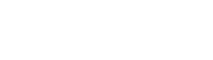# Introduction to Data Visualization

A beginner's guide to data visualization tools and techniques.

## Types of Vizualizations

There are many options when choosing the type of visualization to use. Examples of a few of the most common types are listed below. These examples are generated from data in the popular auto-mpg training data set using the ggplot2 package in R. For examples of the code used to create each visualization, see the "Common Visualization Methods" tab.

## Scatter Plot

Scatter plots are charts depicting at least two sets of plotted data points. Scatter plots show the relationship between the different data sets. Here we see the relationship between overall weight and the mileage per gallon for each vehicle within the auto-mpg training data set.## Line Plot

Similar to scatter plots, line plots also show how two or more values are related. Here we see the same chart used in the scattter plot example displayed as a line plot.## Bar Plot

A bar plot uses rectangular bars to display how large each value is. This example shows the number of each vehicle with the respective number of engine cylinders in the auto-mpg training data set.## Histogram

Similar to a bar plot, a histogram also uses rectangular bars to represent data. However, instead of representing specific amounts, each bar represents a range of data. This example shows the number of cars in the auto-mpg training data set with mileage per gallon rates in ranges of 10. The visualization shows that within the data set, the greatest number of cars achieve between 20 and 30 miles per gallon.## Density Plot

A density plot is a smoother variation of a histogram. The peaks of the density plot show where values are concentrated within an interval range. Here is an example of the same data used to generate the histogram above in the form of a density plot.## Box Plot

Box plots show the spread of data points within a sample of data. The lines outside of the box indicate the lowest and highest value within the range of data points. The line through the center fo the box represents the median for all of the data. A boxplot is divided into four quartiles. A quartile contains a portion of the data within the data set above or below a certain value. The first quartile is the middle value between the lowest value and the median. The second quartile is the median and the third quartile is the middle value between the median and the highest value in the data set. The edges of the box represent the beginning of the 2nd and 3rd quartiles of the data sample. The image below helps illustrate the components of a box plot.